## Cornu Spiral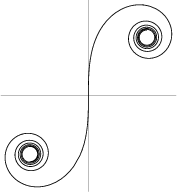A plot in the Complex Plane of the points(1)

whereandare the Fresnel Integrals. The Cornu spiral is also known as the Clothoid or Euler's Spiral. A Cornu spiral describes diffraction from the edge of a half-plane.The Slope of the Cornu spiral(2)

is plotted above.The Slope of the curve's Tangent Vector (above right figure) is(3)

plotted below.The Cesàro Equation for a Cornu spiral is, where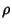is the Radius of Curvature andthe Arc Length. The Torsion is.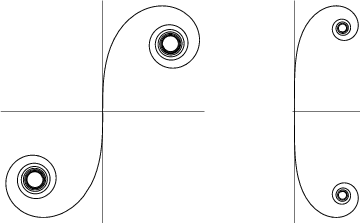Gray (1993) defines a generalization of the Cornu spiral given by parametric equations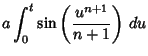(4)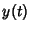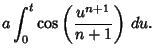(5)The Arc Length, Curvature, and Tangential Angle of this curve are(6)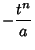(7)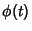(8)

The Cesàro Equation is(9)

Dillen (1990) describes a class of polynomial spirals'' for which the Curvature is a polynomial function of the Arc Length. These spirals are a further generalization of the Cornu spiral.

References

Dillen, F. The Classification of Hypersurfaces of a Euclidean Space with Parallel Higher Fundamental Form.'' Math. Z. 203, 635-643, 1990.

Gray, A. Clothoids.'' §3.6 in Modern Differential Geometry of Curves and Surfaces. Boca Raton, FL: CRC Press, pp. 50-52, 1993.

Lawrence, J. D. A Catalog of Special Plane Curves. New York: Dover, pp. 190-191, 1972.